# Creating a Direct2D game for WinRT

## Posted by Filip Ekberg on 01 Oct 2012

If you want to write a game for Windows 8 and was thinking of using XNA, think again. When creating games for Windows 8 you'll have to go back to using DirectX. But don't worry, with Visual Studio 2012 on Windows 8, you'll get a lot of help doing so. Let's have a look on how to create a Direct2D game for WinRT!

The first thing we would have to do is to fire up Visual Studio and create a new Direct2D project. Can't find it in the C# section for Windows Store applications? That's because we'll have to use C++ for this! You'll find the project template in Other Languages -> Visual C++ -> Windows Store -> Direct2D App (XAML):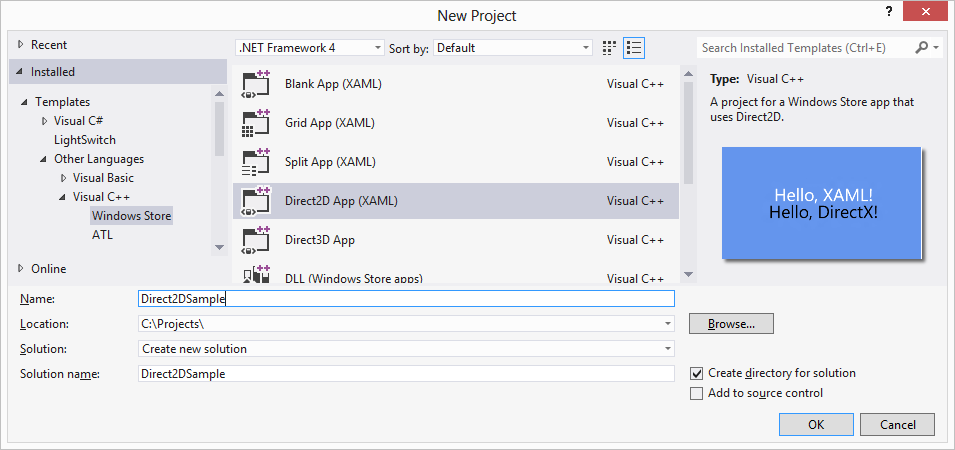When the project is created, we will have a lot of sample files in our solution. As you can see, there are also XAML files in the project! We've got the App.xaml that will tell us which the default view is, which in this case will be DirectXPage.xaml. Let's run it in a simulated mode and see what this default code gives us. With Visual Studio 2012 we get a very handy simulator, to run the application inside it, simply change the target from Local Machine to Simulator: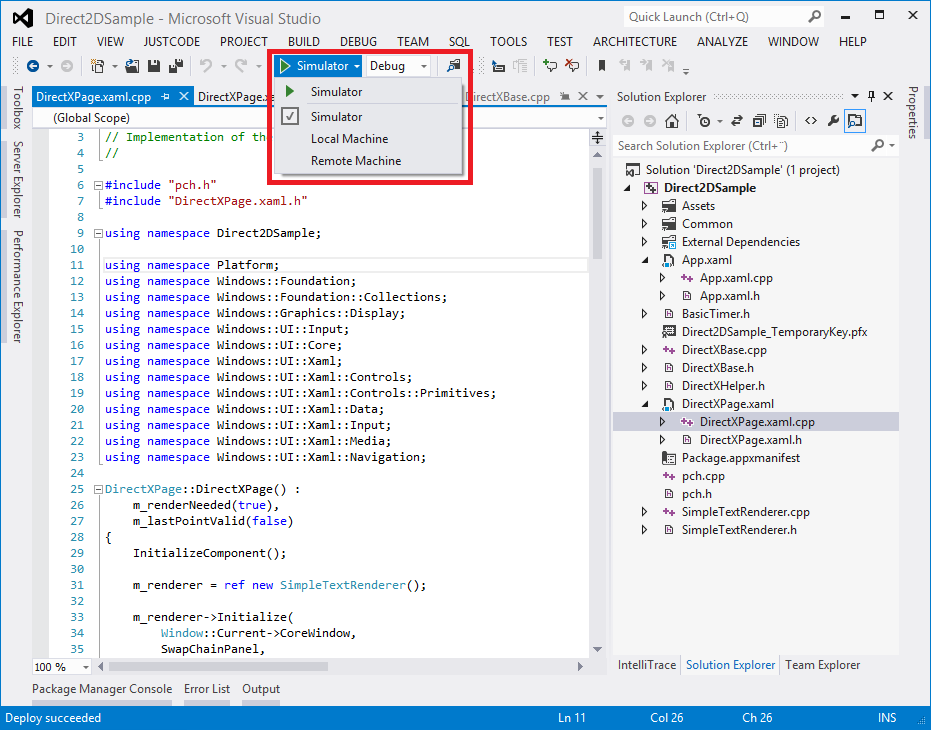This will bring up the simulator and the application that we got by default will let us drag around the title and if we right click the surface, we get an app bar that will allow us to change the background: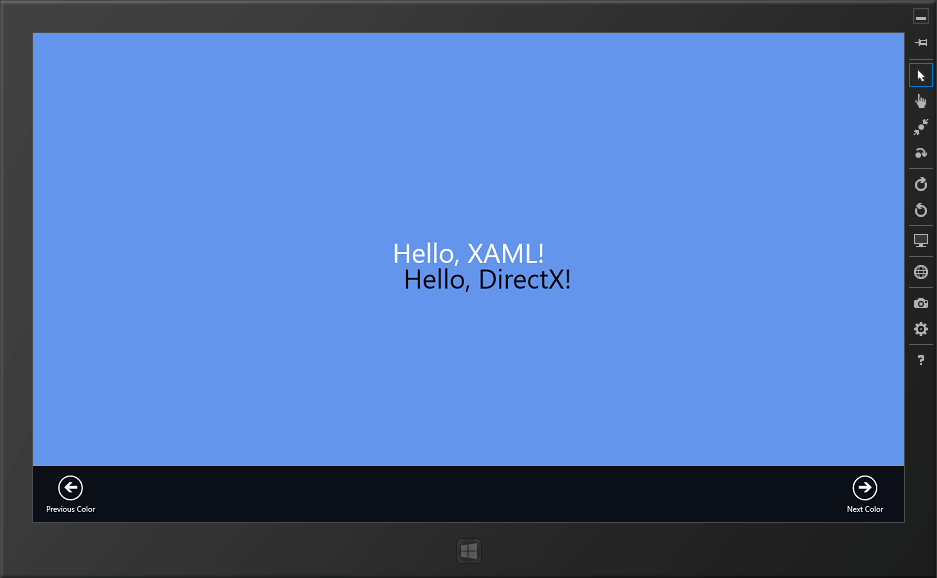Here's a small video on YouTube showing what this looks like when it's running:

What's interesting about this demo is that it combines XAML, C++ and Direct2D. The text in the center that says "Hello, XAML!" is actually just a normal XAML `TextBox`:

``````<TextBlock x:Name="SimpleTextBlock" HorizontalAlignment="Center" FontSize="42" Height="72" Text="Hello, XAML!" Margin="0,0,0,50"/>
``````

The `TextBox` lives inside a content panel called `SwapChainBackgroundPanel` and this is something new. According to MSDN, this panel will only be used when we interop with DirectX:

Implements a XAML layout surface target for Microsoft DirectX interoperation scenarios. This panel has some atypical restrictions on its usage within an app window; see Remarks.

MSDN also says that this only applies to Windows Store applications.

We're getting a lot of DirectX code free here which we don't have to write ourselves. What we do want to take a look at though is the `SimpleTextRenderer`. This class handles drawing the text "Hello, DirectX!" on the screen. It inherits from the `DirectXBase` class which will do all the DirectX magic.

Have a look in the method `SimpleTextRenderer::Render()`, if we were to add a rectangle instead of the text, like this:

``````auto rect = D2D1_RECT_F();
rect.bottom = 100.0f;
rect.top = 0.0f;
rect.left = 0.0f;
rect.right = 100.0f;

m_d2dContext->DrawRectangle(rect, m_blackBrush.Get());
``````

We could run the application and it would look like this: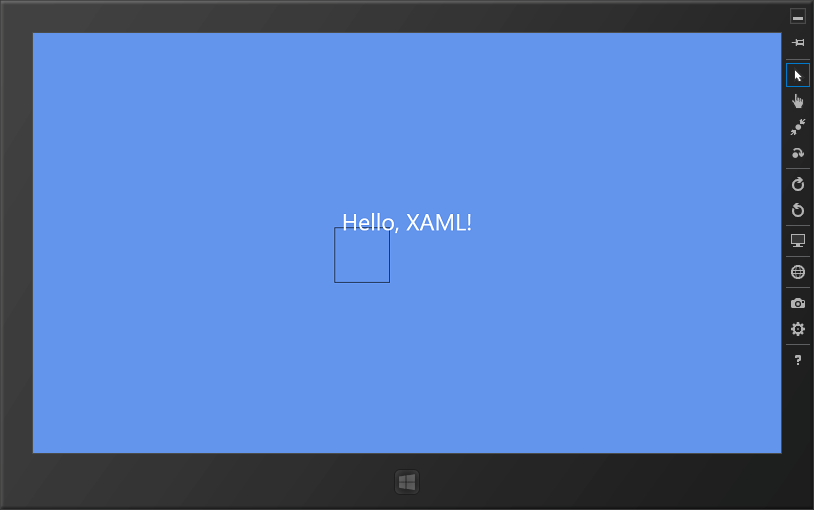Now, try to move the rectangle around, notice that it moves around just like the text we had before did? That's magical! Actually, it's just a transformation going on; the Direct2D context is transformed and the area is moved to wherever the mouse was. These are the lines in that method which changes the transformation:

``````Matrix3x2F translation = Matrix3x2F::Translation(
m_windowBounds.Width / 2.0f - m_textMetrics.widthIncludingTrailingWhitespace / 2.0f + m_textPosition.X,
m_windowBounds.Height / 2.0f - m_textMetrics.height / 2.0f + m_textPosition.Y
);
m_d2dContext->SetTransform(translation * m_orientationTransform2D);
``````

If we simply comment these out, we'll see a completely different result. You should now see the rectangle at the top of the screen being 100 pixels wide and 100 pixels high: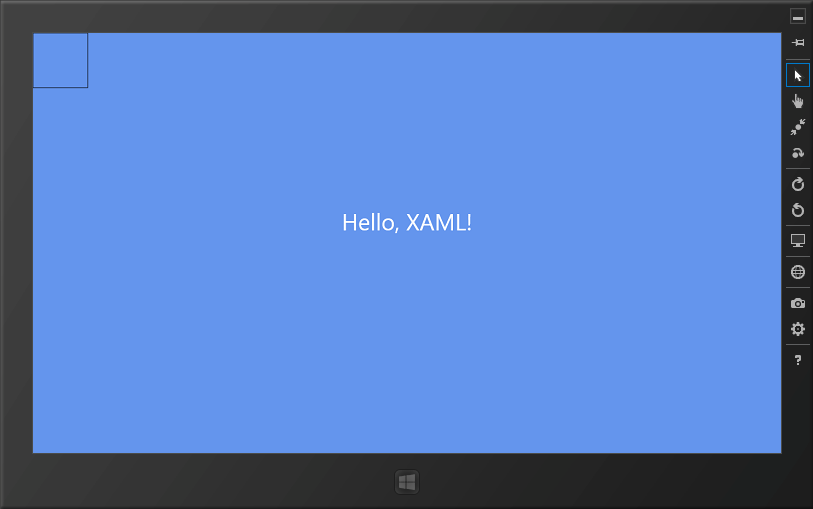Now to make this a bit more interesting, let's make the box move around a little bit. In order for it not to rely on the point & move event, we need to go back to the `DirectXPage` and remove something from the event handler `DirectXPage::OnRendering`. As it is now, it checks if it needs to be rendered by falling back on a boolean variable, but this bool is only set to true when we've moved the mouse (or finger) around. That means that the event handler should look like this instead:

``````void DirectXPage::OnRendering(Object^ sender, Object^ args)
{
m_timer->Update();
m_renderer->Update(m_timer->Total, m_timer->Delta);
m_renderer->Render();
m_renderer->Present();
m_renderNeeded = false;
}
``````

In order for the box to actually move around, we need to change a couple of things in `SimpleTextRenderer::Render` as well as in `SimpleTextRenderer::Update`. First we need to introduce a couple of global variables, I've called them `x` and `y`. These two variables will be in charge of telling us where the rectangle should be drawn on the screen. This means that `SimpleTextRenderer::Render` can look like this:

``````float x = 0.0f;
float y = 0.0f;
void SimpleTextRenderer::Render()
{
m_d2dContext->BeginDraw();

m_d2dContext->Clear(ColorF(BackgroundColors[m_backgroundColorIndex]));

auto rect = D2D1_RECT_F();
rect.top = y;
rect.left = x;

rect.bottom = rect.top + 100.0f;
rect.right = rect.left + 100.0f;

m_d2dContext->DrawRectangle(rect, m_blackBrush.Get());

HRESULT hr = m_d2dContext->EndDraw();
if (hr != D2DERR_RECREATE_TARGET)
{
DX::ThrowIfFailed(hr);
}

m_renderNeeded = false;
}
``````

There's a second pair of global variables that I want to add at this point, these will tell us in which direction the rectangle should be moving. As you might have figured out, I want to make the rectangle bounce around the window!

``````float x_direction = 1.0f;
float y_direction = 1.0f;
``````

Last but not least, I want to make some changes to `SimpleTextRenderer::Update`, since this is where we get information about the delta time, the time since the last frame was updated. Basically I want to increase both `x` and `y` with `timeDelta * 200` and then multiply that with the current direction of which we're moving the rectangle.

When bouncing something around, we need to know the bounds of the window so that the rectangle does not exit outside the window. Luckily for us, the class that we're inheriting from has a member variable called `m_windowBounds` which is a rectangle that tells us the current window bounds.

We can use this rectangle to check if we're outside of the bounds and then we also need to check that we don't reach below 0 in either `x` or `y` direction. This leaves us with a method looking like this:

``````float x_direction = 1.0f;
float y_direction = 1.0f;
void SimpleTextRenderer::Update(float timeTotal, float timeDelta)
{
x += (timeDelta * 200) * x_direction;
y += (timeDelta * 200) * y_direction;

if(x > m_windowBounds.Width - 100.0f || x <= 0) {
y_direction *= 1.0f;
x_direction *= -1.0f;
}
if(y > m_windowBounds.Height - 100.0f || y <= 0) {
y_direction *= -1.0f;
x_direction *= 1.0f;
}
}
``````

Side note: The global variables need to be defined before they're used, add the floats to the top of the file

Here's a video of what this looks like when the application is running inside the Windows 8 Simulator:

Combining XAML with C++ and DirectX will let us create very nice applications and games. Go ahead and great your cool game today and play around with the DirectX samples that come with Visual Studio 2012!